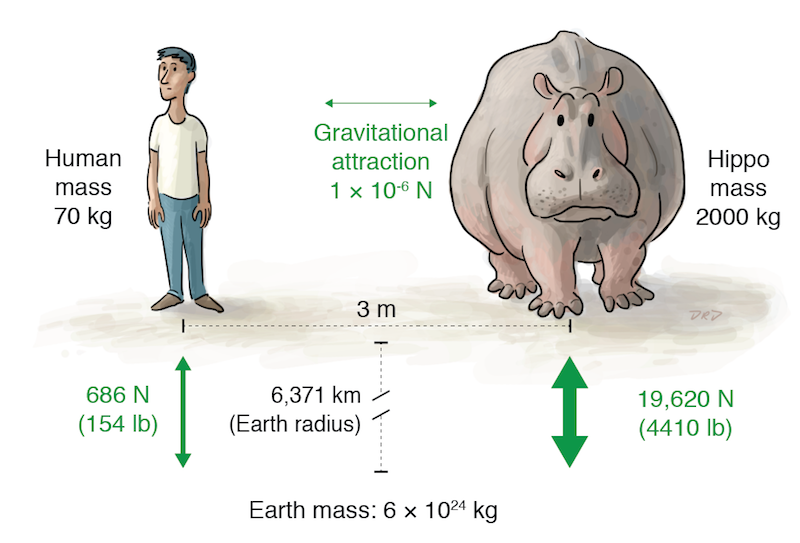Illustrations for a SERP Teacher Tune-up on gravity. The force of gravitational attraction between two objects is proportional the product of their masses divided by the square of the distance between them (measured from the center—hence the distance to the center of Earth in the first illustration). Gravity is an extremely weak force: that negative 6 exponent in the force of attraction between the man and the hippo is telling us their attraction, while real, is too slight to notice.Galileo famously discovered that objects of different masses accelerate at the same rate in free fall. Why is that? Newton, born the year Galileo died, provided the explanation. Mass has two essential features: it is gravitational (mass attracts mass) and it is inertial (mass tends to remain in its state of motion unless acted upon by a force). These two aspects of mass cancel each other when objects fall: it's easier to get the 154 pound man moving than to get the 4410 pound hippo moving, but there is an proportionally smaller gravitational force acting on the man. So free-fall acceleration is always the same. Elegant!The gravitational attraction between two objects is mutual: an apple pulls on Earth just as strongly as Earth pulls on the apple. But Earth has a lot more inertia, so the falling apple does most of the moving. Roller skates and a piece of string make these facts more accessible.